|

# “新零售”时代,净水市场如何“破茧成蝶”?

阿里巴巴集团董事局主席马云在杭州 云栖大会上首次提出“新零售”， 不到一年时间，“新零售”模式已经在中国的各行各业开花结果，净水行业也不例外。

新零售时代到来之际，认清市场的发展方向，针对新的变化调整战略布局是每家企业的生存准则，那么净水新零售时代的变化有哪些？从业者们又该如何改变？

10月12日，由蓝巢国际 直饮水时代主办，国美零售协办的第四届中国净水产业生态峰会在北京新国展举办，峰会以“新零售 聚匠心 源于净”为主题，来自全国的厂家代表、分销商代表等三百多人汇聚一堂，美的、安吉尔、奈固、泉来、A.O.史密斯、沁园等多家企业代表及行业专家纷纷就目前净水市场现状表述了自己观点，成为本届北京国际水展一大亮点。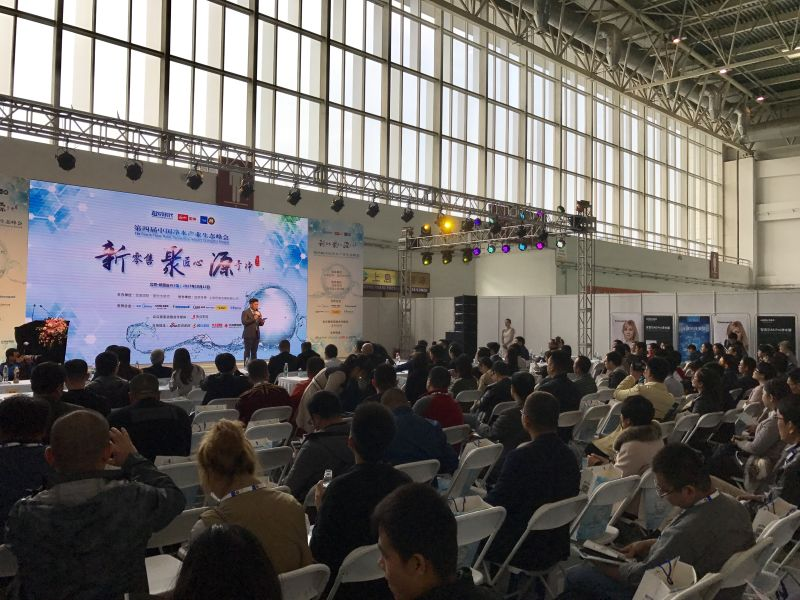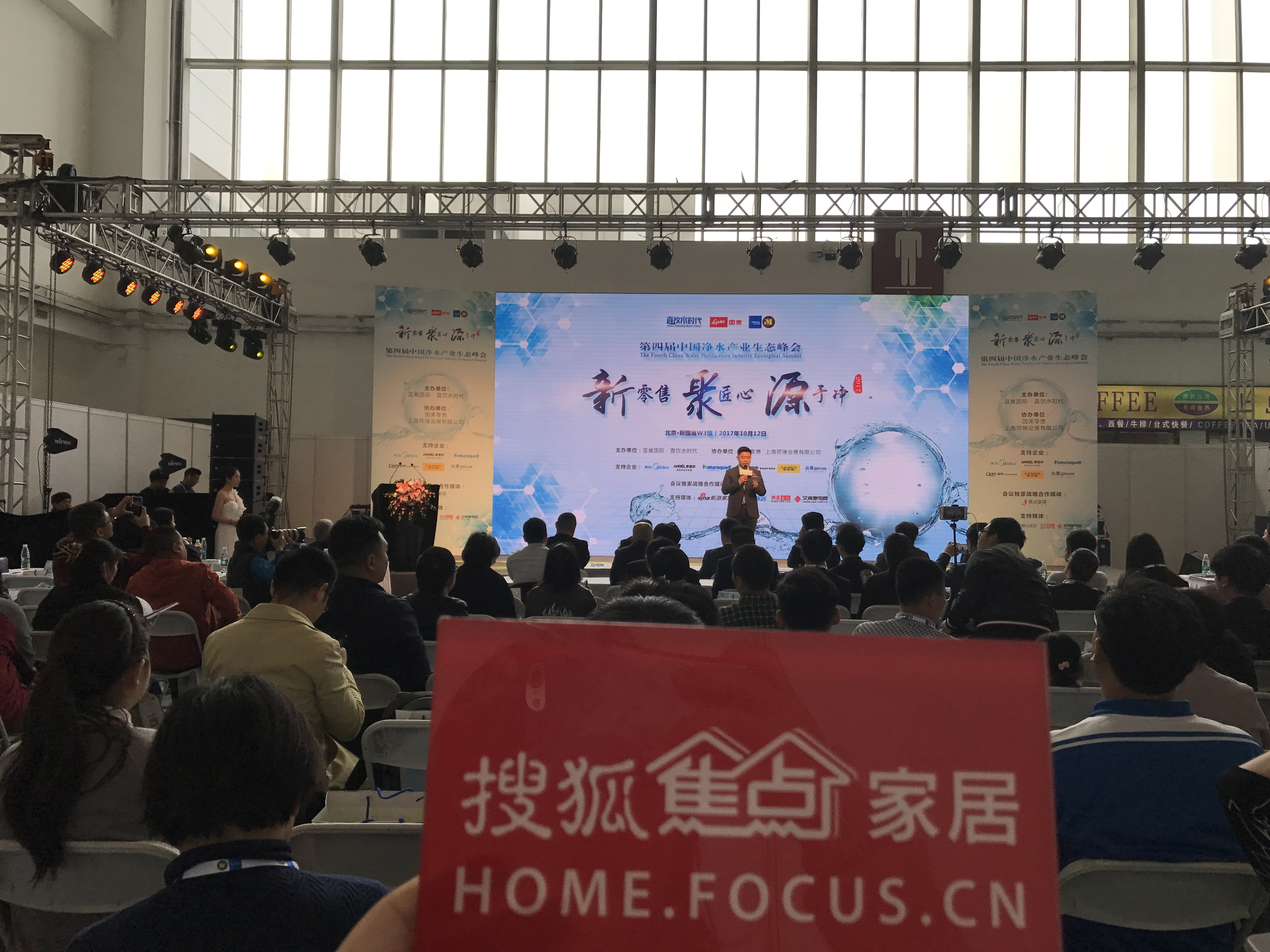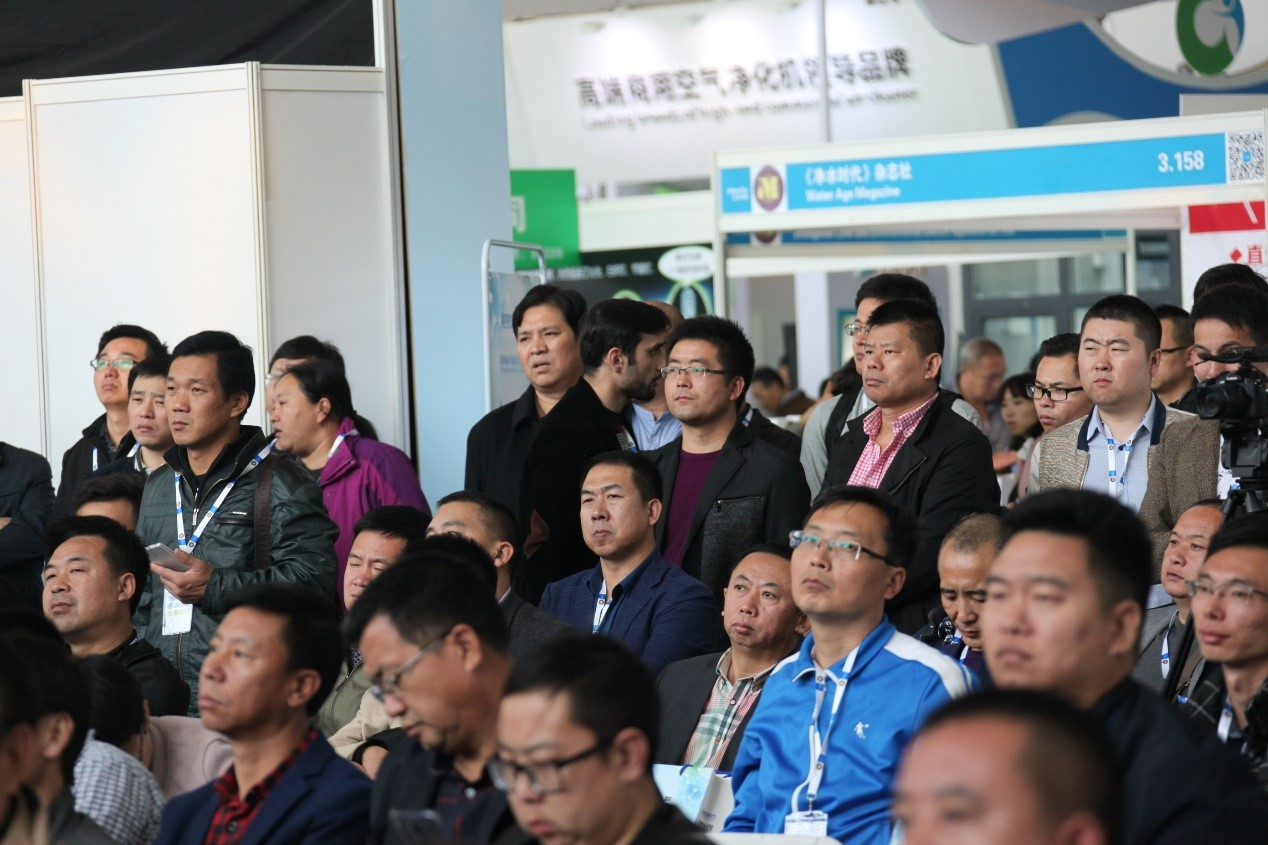企业应有匠心精神

在当前水质状况不容乐观的情况下，健康饮水家电产品已经成为保护人体饮水安全和健康的一道外力屏障。但我国目前净水行业标准尚不完善，消费者对饮用水安全的意识还处于培养阶段，净水器的普及率相比欧美发达国家要低得多，巨大的发展机遇要求净水企业在新零售时代中更好地适应市场规律。

对于此，中国质量万里行促进会副会长兼秘书长高伯海表示，在这个唯快不破的时代，追求快速几乎成为所有企业竞争力的重要途径，但在这样的快车道上，净水企业更应该发挥匠心精神，始终坚持品质第一，在精耕细作中稳健前行。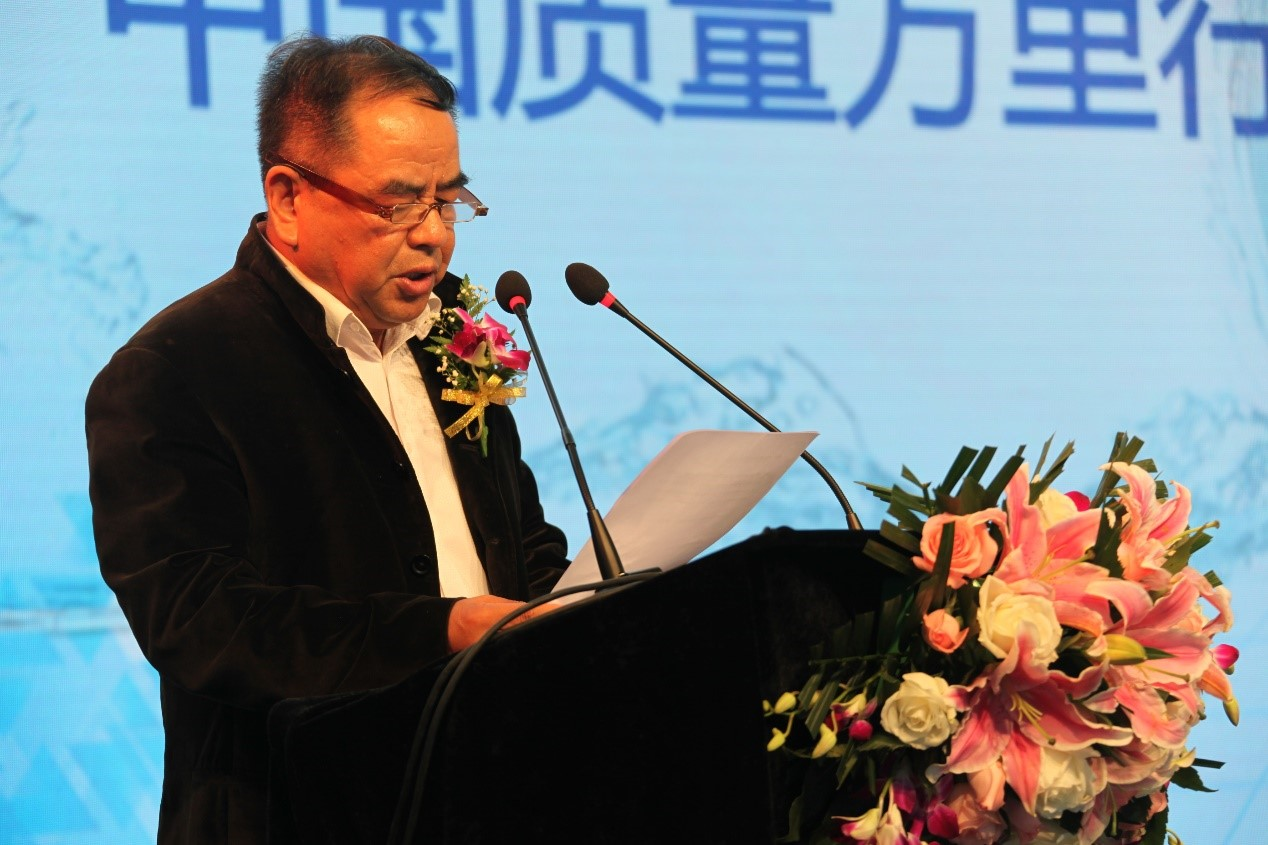众所周知，净水的关键部分是膜芯，要做到安全健康的饮用水，膜芯质量至关重要。中国是个人口大国，同时也是膜大国，应用量非常大，但也同时存在应用层次偏低，应用领域偏窄，膜产业配套不足等问题。中国膜工业协会秘书长王继文强调今后膜协将花更多的精力关注家用净水，为中国的家用净水市场加油助力。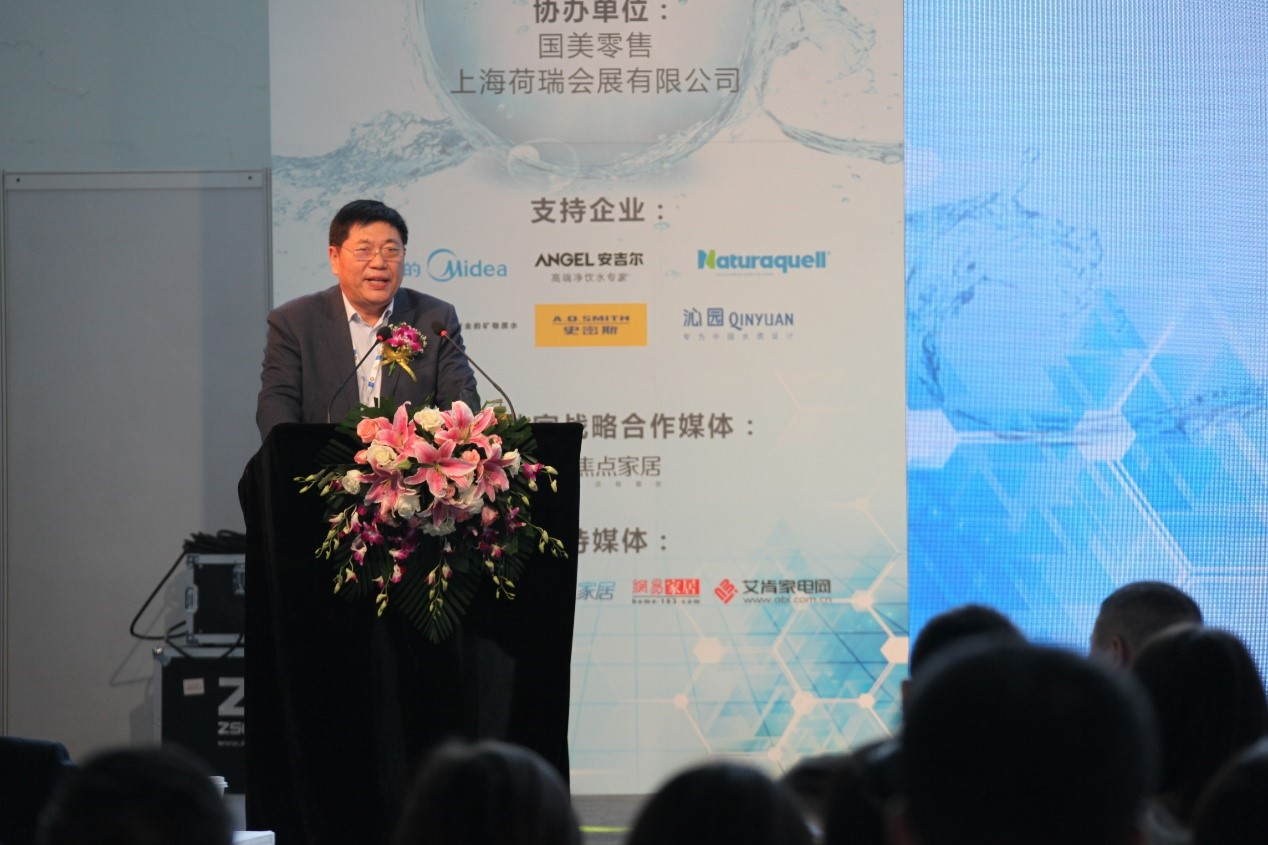中国疾控中心研究所博导、研究员白雪涛先生发表主题演讲。他介绍了安全饮用水方面的知识，并强调净水器的发展方向有四条：时尚简约、安全可靠、节能环保、经济高效，各大净水企业应该努力朝着这四个方面发展。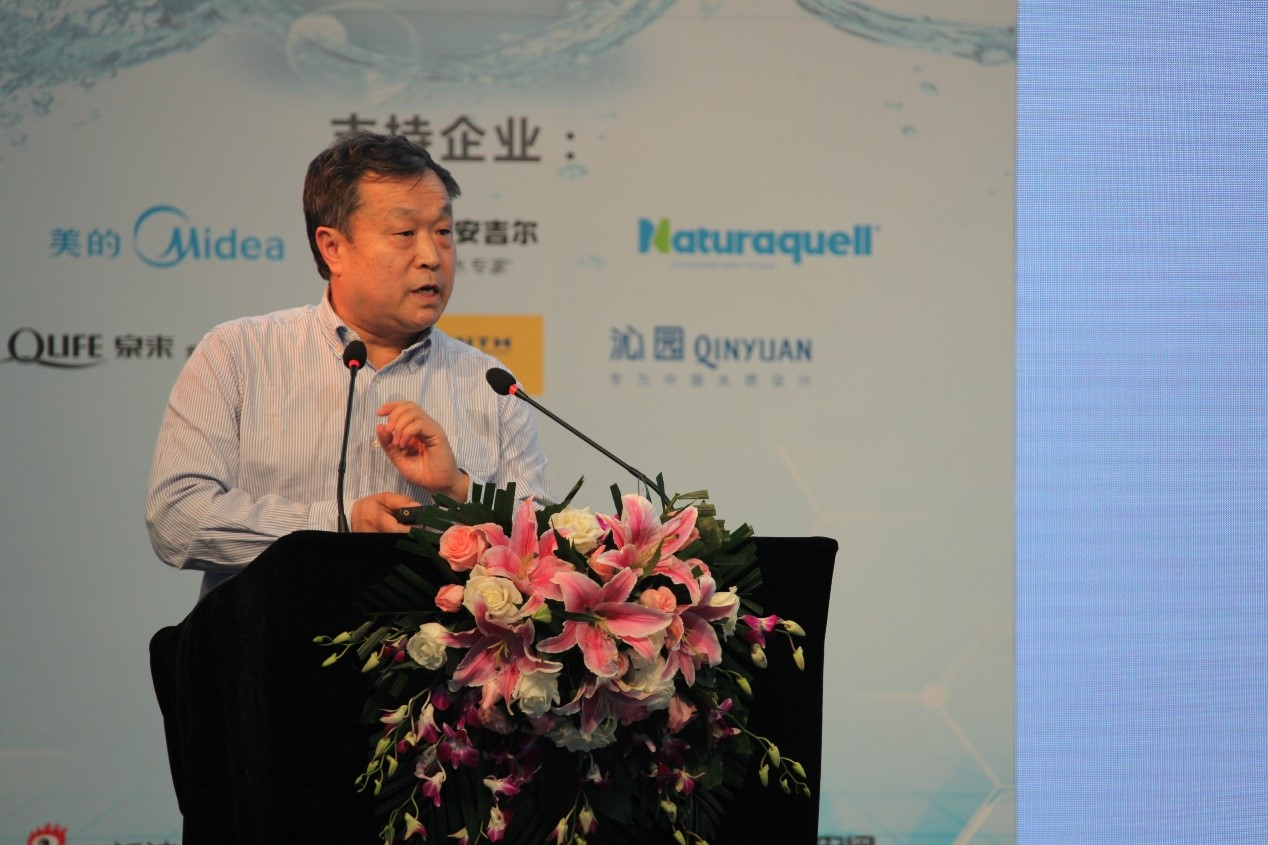芜湖美的厨卫电器制造有限公司净水运营总监张佳龙发表《匠心与责任》主题演讲，他将美的成为净水行业领航者的成绩归于几方面的努力：第一，美的一直以责任为导向，充分挖掘消费者对于净饮水产品的关注点；第二，美的会根据饮水环境的不同不断地进行探索研究，满足消费者的需求。美的坚持以高质量的产品带给消费者一份满意的答卷：凡事以消费者为中心，这样的产品、这样的企业才能够更有效的去服务大众和社会。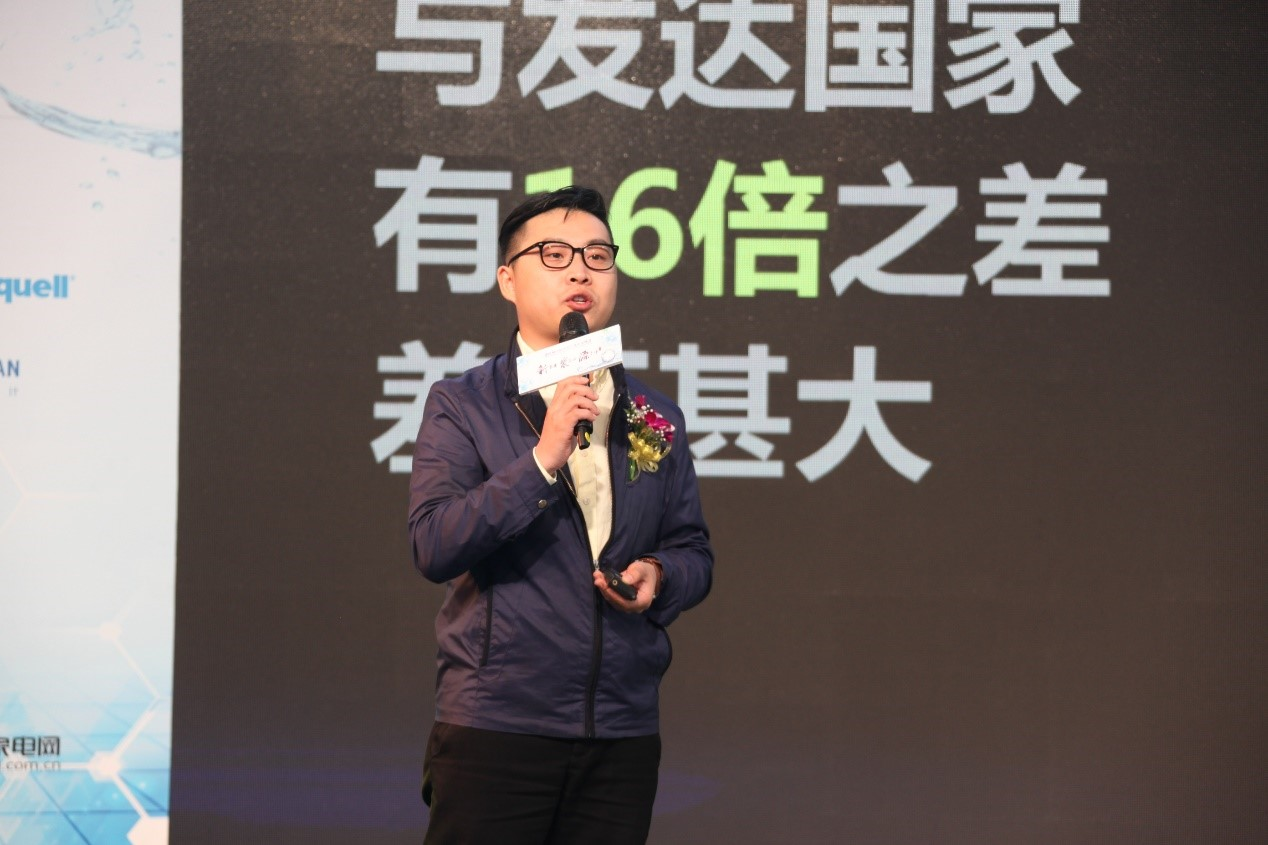净水消费从物质型转向服务型

在此次峰会现场，多位嘉宾在发言过程中提到了净水新零售，何为新零售？随着互联网的发展深化，不只是日用百货、食品、图书，净水的消费场景也发生了巨大变化。深圳安吉尔饮水产业集团营销中心副总经理郝晓伟表示：“不管时代怎么变化，安吉尔最关注的有两件事情：第一是用户的需求到底是什么；第二是商品是否能够满足用户的需求或者是更好的满足用户的需求，产品立于市场就一定要从实际出发。”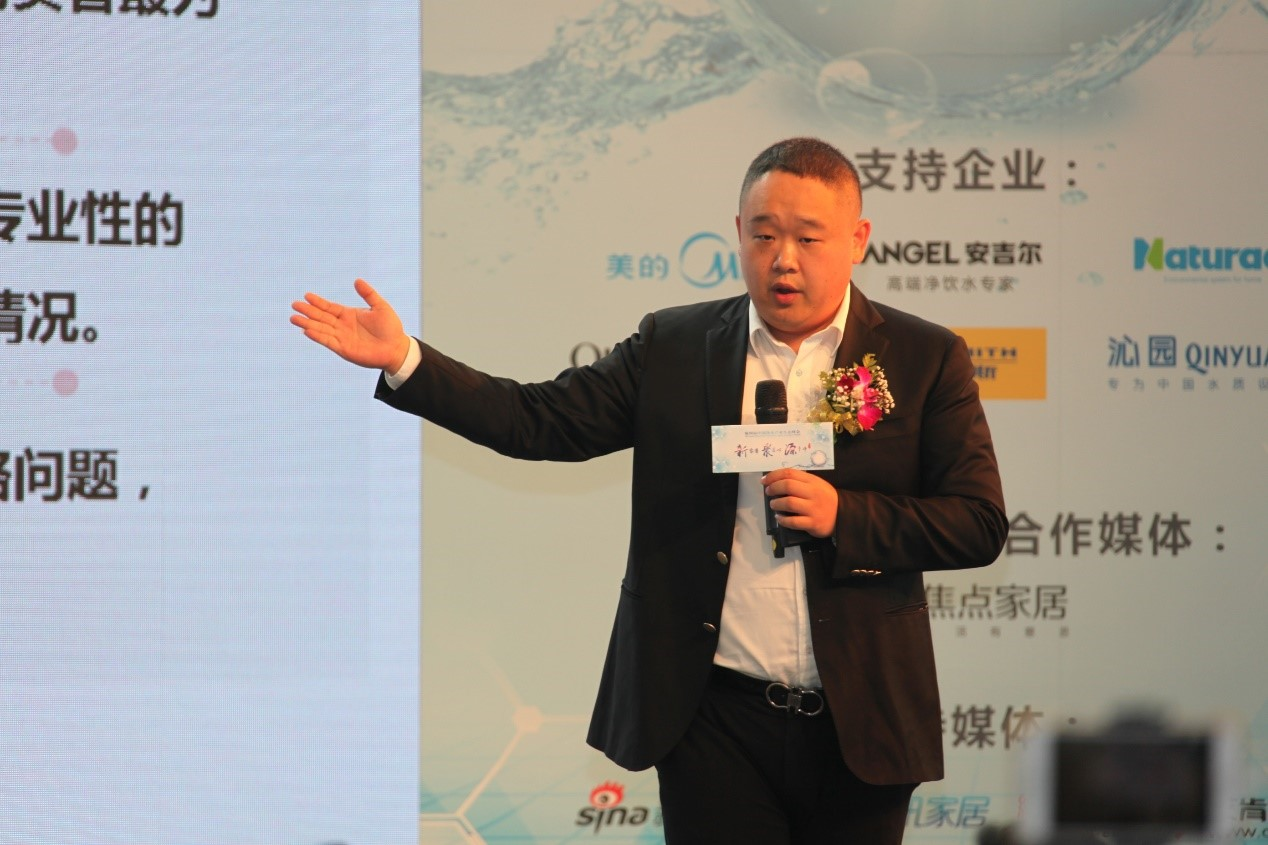奈固（上海）环保科技有限公司总经理孟庆东发表《精品 新零售》主题演讲，他表示新零售和精品的背后其实就是一种匠心的精神，奈固一直秉持着匠心理念，要求每一个产品都做到细致精巧，通过不断深耕去把握产品的质量。“相关数据显示，2017年上半年净水器零售额同比增速达到30.6%，远高于家电行业平均增速，净水市场整体规模仍将继续保持快速增长，但是也面临着新的形势、机遇和挑战。”国美零售控股有限公司生活家电事业部总经理唐佑强表示，对于“新零售”，国美有着自己的独特见解，那就是产品与用户互为前提，即在以用户需求为核心的前提下，继续挖掘用户的深层次需求。净水产业是促进饮水健康安全的关键领域，而新零售是推动净水产业升级发展的助推器。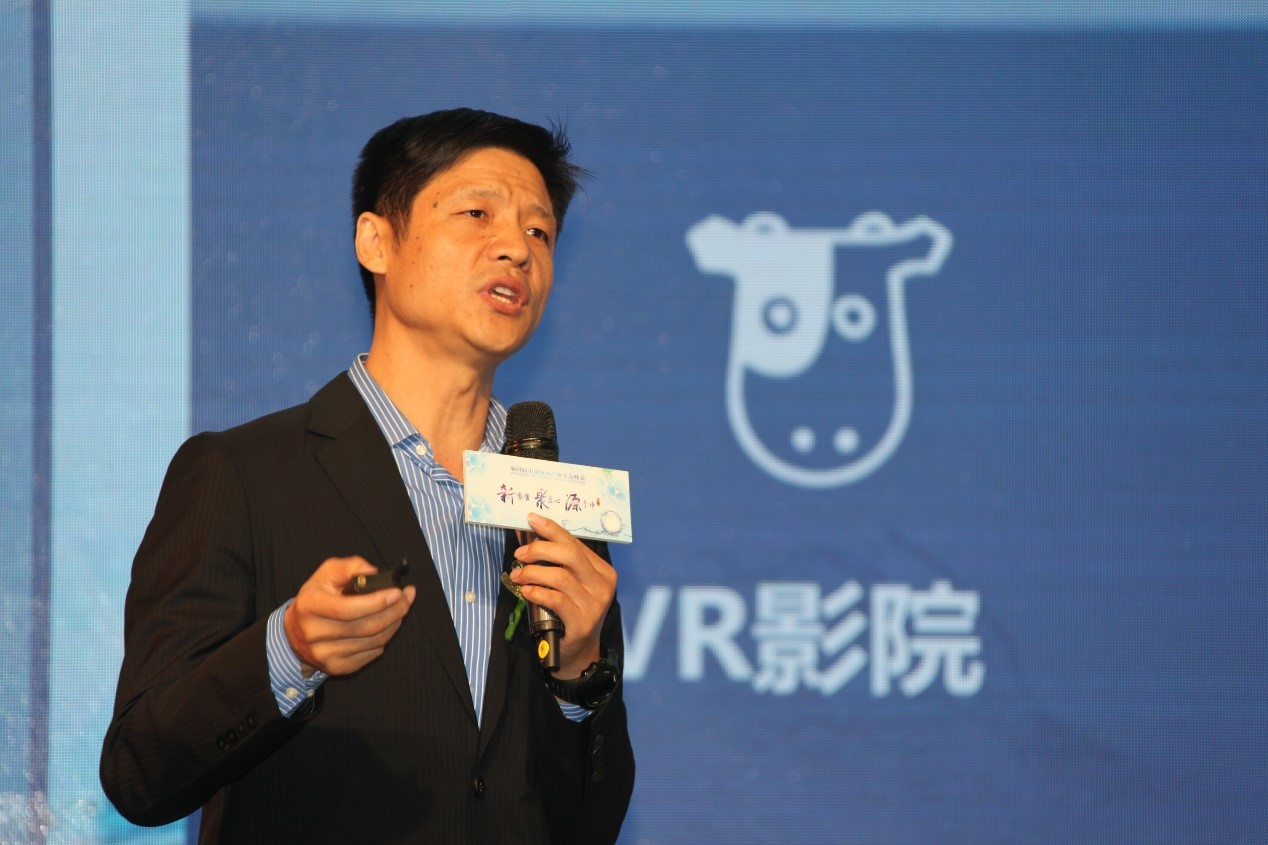商家需要应势而变

中国疾病预防控制中心环境所水质安全研究室主任、研究员，蓝巢国际研究院院长鄂学礼发表《环境健康家电市场洞察》的主题演讲并发布了净水行业配件市场研究报告，报告显示2017年上半年反渗透、纳滤膜整体销售规模达2545万支，其中内销数量为1742万支，出口数量为803万支。家用净水器增压泵上半年整体销售量达1089万支，内销数量为955万支，出口数量为134万支。他认为目前的环境健康家电市场只是集聚没有形成集群，如果形成集群对整个市场技术的提升会有好处，但是在这种情况下来，也促使各个企业在创新方面做了大量的工作，这对配件企业的创新和整个配件品质的提升都起到了一个非常好的作用。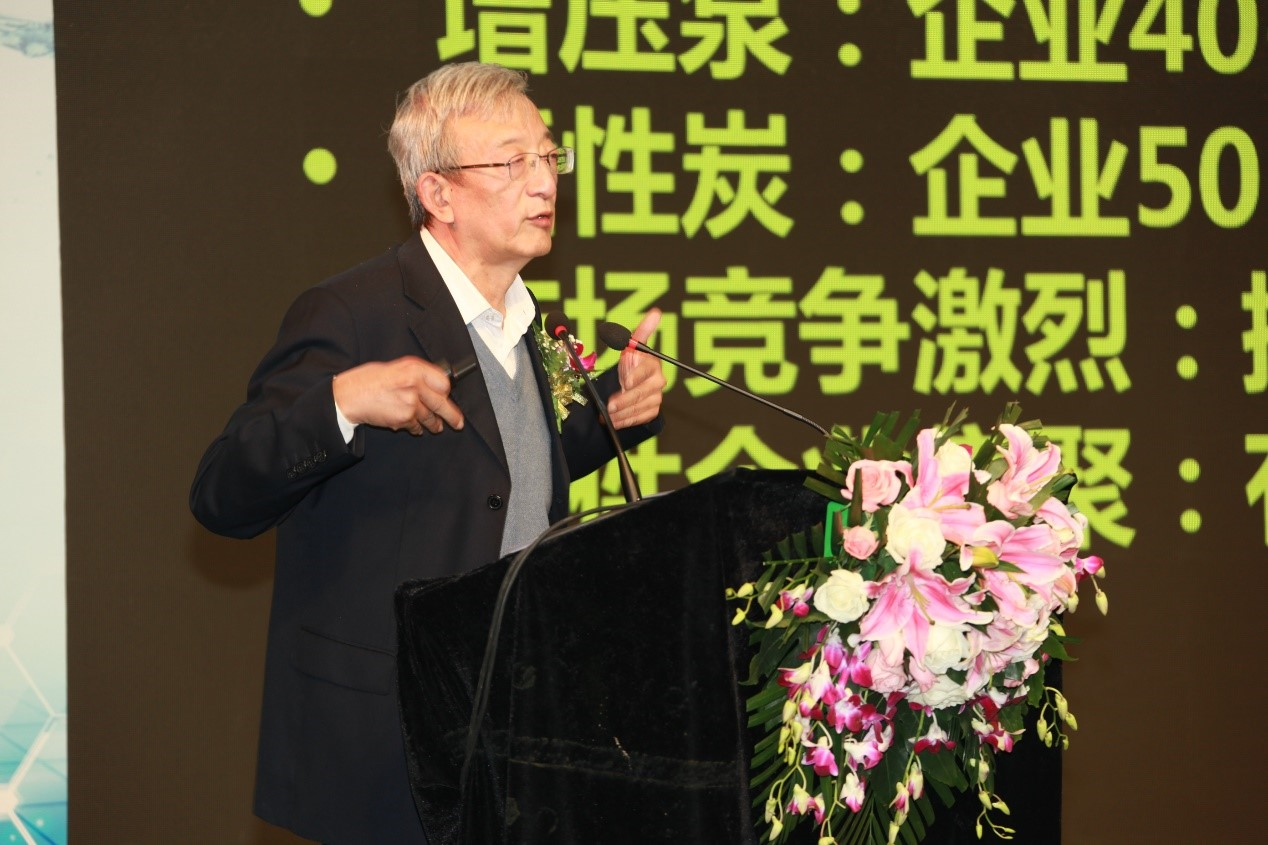中国疾病预防控制中心环境所水质安全研究室主任、研究员，蓝巢国际研究院院长 鄂学礼

北京博信达商贸有限公司副总经理王军发表主题演讲。他详细地介绍了博信达在经营管理过程中的经验，他认为新零售时代商家需应势而变，掌握和运用客户的信息，了解客户的习惯，充分挖掘客户的需求。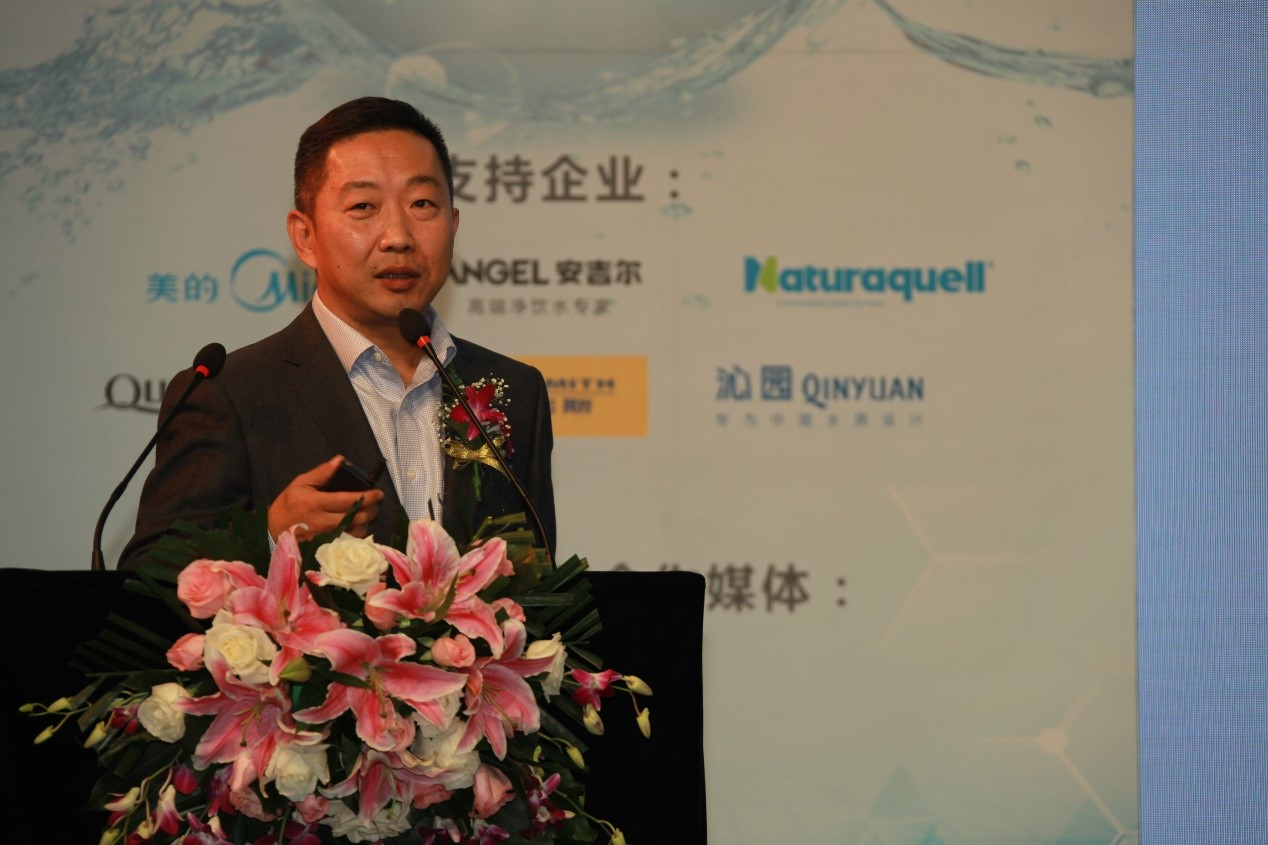北京羽隆万源贸易有限公司的总经理李连文也在峰会现场分享了经验，他强调在开展新零售之前要有新思维，没有这个新的思维就不会出现新零售。

会议的最后，组委会为获奖企业颁奖，其中安吉尔、美的、奈固获得“2017净水行业产品匠心奖”，美的、安吉尔、史密斯、泉来荣获“2017净水行业品牌影响力奖”。与此同时，第二届国美全民净水节也在此次峰会上启动。

`声明：本文由入驻焦点开放平台的作者撰写，除焦点官方账号外，观点仅代表作者本人，不代表焦点立场错误信息举报电话： 400-099-0099，邮箱：jubao@vip.sohu.com，或点此进行意见反馈，或点此进行举报投诉。`A B C D E F G H J K L M N P Q R S T W X Y Z
A - B - C - D - E
• A
• 鞍山
• 安庆
• 安阳
• 安顺
• 安康
• 澳门
• B
• 北京
• 保定
• 包头
• 巴彦淖尔
• 本溪
• 蚌埠
• 亳州
• 滨州
• 北海
• 百色
• 巴中
• 毕节
• 保山
• 宝鸡
• 白银
• 巴州
• C
• 承德
• 沧州
• 长治
• 赤峰
• 朝阳
• 长春
• 常州
• 滁州
• 池州
• 长沙
• 常德
• 郴州
• 潮州
• 崇左
• 重庆
• 成都
• 楚雄
• 昌都
• 慈溪
• 常熟
• D
• 大同
• 大连
• 丹东
• 大庆
• 东营
• 德州
• 东莞
• 德阳
• 达州
• 大理
• 德宏
• 定西
• 儋州
• 东平
• E
• 鄂尔多斯
• 鄂州
• 恩施
F - G - H - I - J
• F
• 抚顺
• 阜新
• 阜阳
• 福州
• 抚州
• 佛山
• 防城港
• G
• 赣州
• 广州
• 桂林
• 贵港
• 广元
• 广安
• 贵阳
• 固原
• H
• 邯郸
• 衡水
• 呼和浩特
• 呼伦贝尔
• 葫芦岛
• 哈尔滨
• 黑河
• 淮安
• 杭州
• 湖州
• 合肥
• 淮南
• 淮北
• 黄山
• 菏泽
• 鹤壁
• 黄石
• 黄冈
• 衡阳
• 怀化
• 惠州
• 河源
• 贺州
• 河池
• 海口
• 红河
• 汉中
• 海东
• 怀来
• I
• J
• 晋中
• 锦州
• 吉林
• 鸡西
• 佳木斯
• 嘉兴
• 金华
• 景德镇
• 九江
• 吉安
• 济南
• 济宁
• 焦作
• 荆门
• 荆州
• 江门
• 揭阳
• 金昌
• 酒泉
• 嘉峪关
K - L - M - N - P
• K
• 开封
• 昆明
• 昆山
• L
• 廊坊
• 临汾
• 辽阳
• 连云港
• 丽水
• 六安
• 龙岩
• 莱芜
• 临沂
• 聊城
• 洛阳
• 漯河
• 娄底
• 柳州
• 来宾
• 泸州
• 乐山
• 六盘水
• 丽江
• 临沧
• 拉萨
• 林芝
• 兰州
• 陇南
• M
• 牡丹江
• 马鞍山
• 茂名
• 梅州
• 绵阳
• 眉山
• N
• 南京
• 南通
• 宁波
• 南平
• 宁德
• 南昌
• 南阳
• 南宁
• 内江
• 南充
• P
• 盘锦
• 莆田
• 平顶山
• 濮阳
• 攀枝花
• 普洱
• 平凉
Q - R - S - T - W
• Q
• 秦皇岛
• 齐齐哈尔
• 衢州
• 泉州
• 青岛
• 清远
• 钦州
• 黔南
• 曲靖
• 庆阳
• R
• 日照
• 日喀则
• S
• 石家庄
• 沈阳
• 双鸭山
• 绥化
• 上海
• 苏州
• 宿迁
• 绍兴
• 宿州
• 三明
• 上饶
• 三门峡
• 商丘
• 十堰
• 随州
• 邵阳
• 韶关
• 深圳
• 汕头
• 汕尾
• 三亚
• 三沙
• 遂宁
• 山南
• 商洛
• 石嘴山
• T
• 天津
• 唐山
• 太原
• 通辽
• 铁岭
• 泰州
• 台州
• 铜陵
• 泰安
• 铜仁
• 铜川
• 天水
• 天门
• W
• 乌海
• 乌兰察布
• 无锡
• 温州
• 芜湖
• 潍坊
• 威海
• 武汉
• 梧州
• 渭南
• 武威
• 吴忠
• 乌鲁木齐
X - Y - Z
• X
• 邢台
• 徐州
• 宣城
• 厦门
• 新乡
• 许昌
• 信阳
• 襄阳
• 孝感
• 咸宁
• 湘潭
• 湘西
• 西双版纳
• 西安
• 咸阳
• 西宁
• 仙桃
• 西昌
• Y
• 运城
• 营口
• 盐城
• 扬州
• 鹰潭
• 宜春
• 烟台
• 宜昌
• 岳阳
• 益阳
• 永州
• 阳江
• 云浮
• 玉林
• 宜宾
• 雅安
• 玉溪
• 延安
• 榆林
• 银川
• Z
• 张家口
• 镇江
• 舟山
• 漳州
• 淄博
• 枣庄
• 郑州
• 周口
• 驻马店
• 株洲
• 张家界
• 珠海
• 湛江
• 肇庆
• 中山
• 自贡
• 资阳
• 遵义
• 昭通
• 张掖
• 中卫

1室1厅1厨1卫1阳台

1
2
3
4
5

0
1
2

1

1

0
1
2
3报名成功，资料已提交审核A B C D E F G H J K L M N P Q R S T W X Y Z
A - B - C - D - E
• A
• 鞍山
• 安庆
• 安阳
• 安顺
• 安康
• 澳门
• B
• 北京
• 保定
• 包头
• 巴彦淖尔
• 本溪
• 蚌埠
• 亳州
• 滨州
• 北海
• 百色
• 巴中
• 毕节
• 保山
• 宝鸡
• 白银
• 巴州
• C
• 承德
• 沧州
• 长治
• 赤峰
• 朝阳
• 长春
• 常州
• 滁州
• 池州
• 长沙
• 常德
• 郴州
• 潮州
• 崇左
• 重庆
• 成都
• 楚雄
• 昌都
• 慈溪
• 常熟
• D
• 大同
• 大连
• 丹东
• 大庆
• 东营
• 德州
• 东莞
• 德阳
• 达州
• 大理
• 德宏
• 定西
• 儋州
• 东平
• E
• 鄂尔多斯
• 鄂州
• 恩施
F - G - H - I - J
• F
• 抚顺
• 阜新
• 阜阳
• 福州
• 抚州
• 佛山
• 防城港
• G
• 赣州
• 广州
• 桂林
• 贵港
• 广元
• 广安
• 贵阳
• 固原
• H
• 邯郸
• 衡水
• 呼和浩特
• 呼伦贝尔
• 葫芦岛
• 哈尔滨
• 黑河
• 淮安
• 杭州
• 湖州
• 合肥
• 淮南
• 淮北
• 黄山
• 菏泽
• 鹤壁
• 黄石
• 黄冈
• 衡阳
• 怀化
• 惠州
• 河源
• 贺州
• 河池
• 海口
• 红河
• 汉中
• 海东
• 怀来
• I
• J
• 晋中
• 锦州
• 吉林
• 鸡西
• 佳木斯
• 嘉兴
• 金华
• 景德镇
• 九江
• 吉安
• 济南
• 济宁
• 焦作
• 荆门
• 荆州
• 江门
• 揭阳
• 金昌
• 酒泉
• 嘉峪关
K - L - M - N - P
• K
• 开封
• 昆明
• 昆山
• L
• 廊坊
• 临汾
• 辽阳
• 连云港
• 丽水
• 六安
• 龙岩
• 莱芜
• 临沂
• 聊城
• 洛阳
• 漯河
• 娄底
• 柳州
• 来宾
• 泸州
• 乐山
• 六盘水
• 丽江
• 临沧
• 拉萨
• 林芝
• 兰州
• 陇南
• M
• 牡丹江
• 马鞍山
• 茂名
• 梅州
• 绵阳
• 眉山
• N
• 南京
• 南通
• 宁波
• 南平
• 宁德
• 南昌
• 南阳
• 南宁
• 内江
• 南充
• P
• 盘锦
• 莆田
• 平顶山
• 濮阳
• 攀枝花
• 普洱
• 平凉
Q - R - S - T - W
• Q
• 秦皇岛
• 齐齐哈尔
• 衢州
• 泉州
• 青岛
• 清远
• 钦州
• 黔南
• 曲靖
• 庆阳
• R
• 日照
• 日喀则
• S
• 石家庄
• 沈阳
• 双鸭山
• 绥化
• 上海
• 苏州
• 宿迁
• 绍兴
• 宿州
• 三明
• 上饶
• 三门峡
• 商丘
• 十堰
• 随州
• 邵阳
• 韶关
• 深圳
• 汕头
• 汕尾
• 三亚
• 三沙
• 遂宁
• 山南
• 商洛
• 石嘴山
• T
• 天津
• 唐山
• 太原
• 通辽
• 铁岭
• 泰州
• 台州
• 铜陵
• 泰安
• 铜仁
• 铜川
• 天水
• 天门
• W
• 乌海
• 乌兰察布
• 无锡
• 温州
• 芜湖
• 潍坊
• 威海
• 武汉
• 梧州
• 渭南
• 武威
• 吴忠
• 乌鲁木齐
X - Y - Z
• X
• 邢台
• 徐州
• 宣城
• 厦门
• 新乡
• 许昌
• 信阳
• 襄阳
• 孝感
• 咸宁
• 湘潭
• 湘西
• 西双版纳
• 西安
• 咸阳
• 西宁
• 仙桃
• 西昌
• Y
• 运城
• 营口
• 盐城
• 扬州
• 鹰潭
• 宜春
• 烟台
• 宜昌
• 岳阳
• 益阳
• 永州
• 阳江
• 云浮
• 玉林
• 宜宾
• 雅安
• 玉溪
• 延安
• 榆林
• 银川
• Z
• 张家口
• 镇江
• 舟山
• 漳州
• 淄博
• 枣庄
• 郑州
• 周口
• 驻马店
• 株洲
• 张家界
• 珠海
• 湛江
• 肇庆
• 中山
• 自贡
• 资阳
• 遵义
• 昭通
• 张掖
• 中卫• 手机• 分享
• 设计
免费设计
• 计算器
装修计算器
• 入驻
合作入驻
• 联系
联系我们
• 置顶
返回顶部